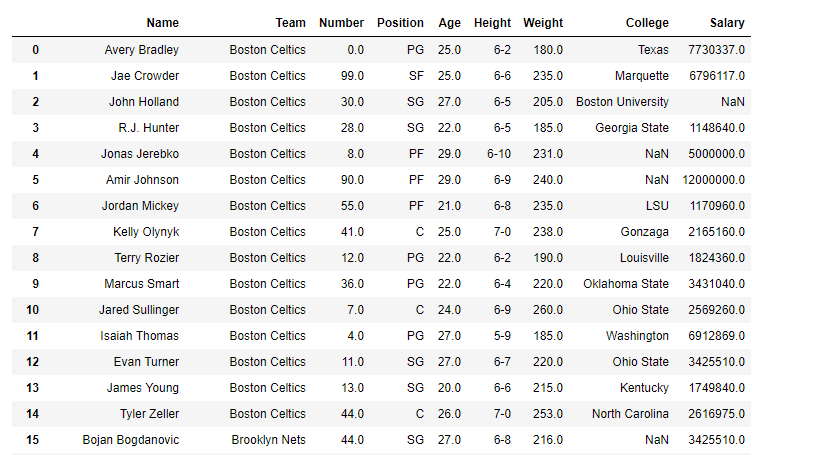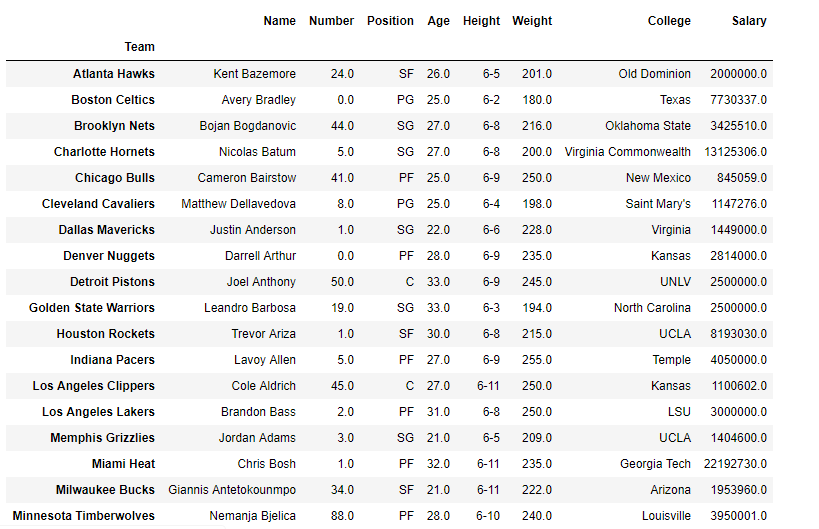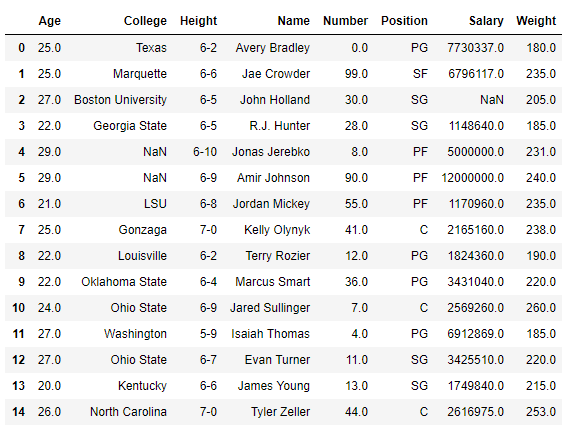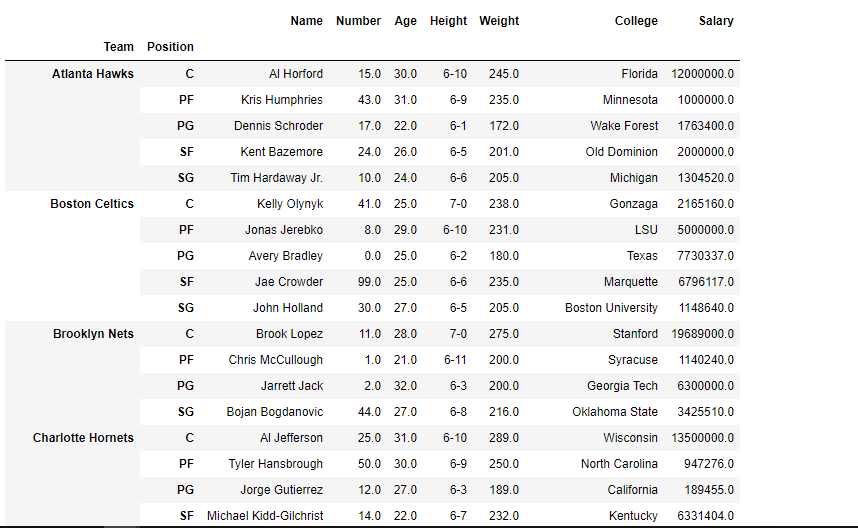GeeksforGeeks App
Open AppBrowser
Continue

# Pandas dataframe.groupby() Method

Pandas groupby is used for grouping the data according to the categories and applying a function to the categories. It also helps to aggregate data efficiently. The Pandas groupby() is a very powerful function with a lot of variations. It makes the task of splitting the Dataframe over some criteria really easy and efficient.

## Pandas dataframe.groupby()

Pandas dataframe.groupby() function is used to split the data into groups based on some criteria. Pandas objects can be split on any of their axes. The abstract definition of grouping is to provide a mapping of labels to group names.

Syntax: DataFrame.groupby(by=None, axis=0, level=None, as_index=True, sort=True, group_keys=True, squeeze=False, **kwargs)

Parameters :

• by : mapping, function, str, or iterable
• axis : int, default 0
• level : If the axis is a MultiIndex (hierarchical), group by a particular level or levels
• as_index : For aggregated output, return object with group labels as the index. Only relevant for DataFrame input. as_index=False is effectively “SQL-style” grouped output
• sort : Sort group keys. Get better performance by turning this off. Note this does not influence the order of observations within each group. groupby preserves the order of rows within each group.
• group_keys : When calling apply, add group keys to index to identify pieces
• squeeze : Reduce the dimensionality of the return type if possible, otherwise return a consistent type

Returns : GroupBy object

Example 1: Use groupby() function to group the data based on the “Team”.

## Python3

 `# importing pandas as pd``import` `pandas as pd` `# Creating the dataframe``df ``=` `pd.read_csv(``"nba.csv"``)` `# Print the dataframe``df`

Output:Now apply the groupby() function.

## Python3

 `# applying groupby() function to``# group the data on team value.``gk ``=` `df.groupby(``'Team'``)` `# Let's print the first entries``# in all the groups formed.``gk.first()`

Output :Let’s print the value contained in any one of the groups. For that use the name of the team. We use the function get_group() to find the entries contained in any of the groups.

## Python3

 `# Finding the values contained in the "Boston Celtics" group``gk.get_group(``'Boston Celtics'``)`

Output :Example 2: Use groupby() function to form groups based on more than one category (i.e. Use more than one column to perform the splitting).

## Python3

 `# importing pandas as pd``import` `pandas as pd` `# Creating the dataframe``df ``=` `pd.read_csv(``"nba.csv"``)` `# First grouping based on "Team"``# Within each team we are grouping based on "Position"``gkk ``=` `df.groupby([``'Team'``, ``'Position'``])` `# Print the first value in each group``gkk.first()`

Output :My Personal Notes arrow_drop_up